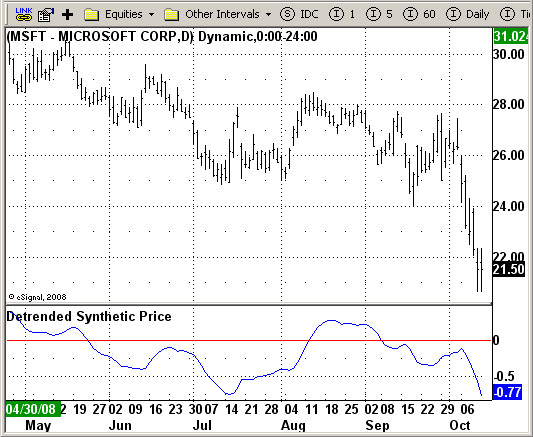# D_DSP (Detrended Synthetic Price)

ICE Data Services -

D_DSP.efs

File Name: D_DSP.efs

Description:
This Indicator plots D_DSP (Detrended Synthetic Price) indicator

Formula Parameters:

• Length: 14

Notes:
Detrended Synthetic Price is a function that is in phase with the dominant cycle of real price data. This DSP is computed by subtracting a half-cycle exponential moving average (EMA) from the quarter cycle
exponential moving average.
See "MESA and Trading Market Cycles" by John Ehlers pages 64 - 70.

D_DSP.efsEFS Code:

```/*********************************

Provided By:

eSignal (Copyright c eSignal), a division of Interactive Data
Formula Script (EFS) is for educational purposes only and may be
modified and saved under a new file name.  eSignal is not responsible
for the functionality once modified.  eSignal reserves the right
to modify and overwrite this EFS file with each new release.

Description:

This Indicator plots D_DSP (Detrended Synthetic Price) indicator

Version:            1.0  09/30/2008

Notes:

Detrended Synthetic Price is a function that is in phase with the
dominant cycle of real price data. This DSP is computed by subtracting
a half-cycle exponential moving average (EMA) from the quarter cycle
exponential moving average.

See "MESA and Trading Market Cycles" by John Ehlers pages 64 - 70.

Formula Parameters:                     Default:

Length                              14

**********************************/

var fpArray = new Array();

function preMain() {
setPriceStudy(false);
setStudyTitle("Detrended Synthetic Price");
setCursorLabelName("D-DSP", 0);
setDefaultBarFgColor(Color.blue, 0);

var x=0;
fpArray[x] = new FunctionParameter("Length", FunctionParameter.NUMBER);
with(fpArray[x++]) {
setLowerLimit(2);
setDefault(14);
}
}

var bInit = false;
var xRes   = null;

function main(Length) {
var nBarState = getBarState();
if (nBarState == BARSTATE_ALLBARS) {
if (Length == null) Length = 14;
}

if (bInit == false) {
xRes = efsInternal("calc", Length, inv("d"));
bInit = true;
}
return xRes.getValue(0);
}

var xInit = false;
var xPrice = null;
var xEMA1 = null;
var xEMA2 = null;

function calc(Length, Interval) {
if(xInit == false) {
xPrice = hl2();
xEMA1 = ema(Length, xPrice);
xEMA2 = ema(Length*2, xPrice);
xInit = true;
}
var nEMA1 = xEMA1.getValue(-1);
var nEMA2 = xEMA2.getValue(-1);
if(nEMA1 == null || nEMA2 == null) return;
return nEMA1 - nEMA2;
}```